# US20060180473A1 - Water energy generator - Google Patents

## Info

Publication number
US20060180473A1
US20060180473A1 US11/060,037 US6003705A US2006180473A1 US 20060180473 A1 US20060180473 A1 US 20060180473A1 US 6003705 A US6003705 A US 6003705A US 2006180473 A1 US2006180473 A1 US 2006180473A1
Authority
US
United States
Prior art keywords
energy
hyperspace
generator
water
coil
Prior art date
Legal status (The legal status is an assumption and is not a legal conclusion. Google has not performed a legal analysis and makes no representation as to the accuracy of the status listed.)
Abandoned
Application number
US11/060,037
Inventor
John St. Clair
Current Assignee (The listed assignees may be inaccurate. Google has not performed a legal analysis and makes no representation or warranty as to the accuracy of the list.)
Individual
Original Assignee
Individual
Priority date (The priority date is an assumption and is not a legal conclusion. Google has not performed a legal analysis and makes no representation as to the accuracy of the date listed.)
Filing date
Publication date
Application filed by Individual filed Critical Individual
Priority to US11/060,037 priority Critical patent/US20060180473A1/en
Publication of US20060180473A1 publication Critical patent/US20060180473A1/en
Abandoned legal-status Critical Current

## Images

•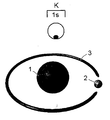•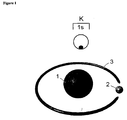•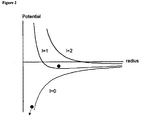•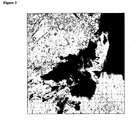•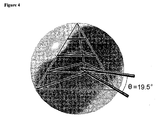•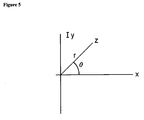•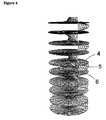•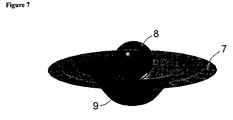•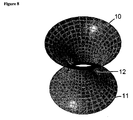•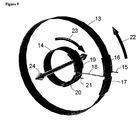••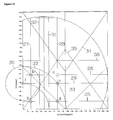•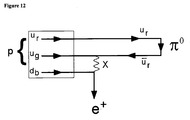•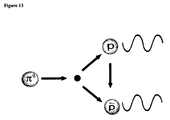•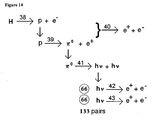•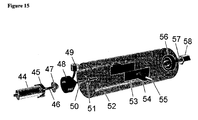•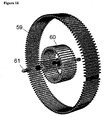•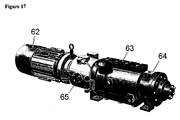## Classifications

• BPERFORMING OPERATIONS; TRANSPORTING
• B64AIRCRAFT; AVIATION; COSMONAUTICS
• B64GCOSMONAUTICS; VEHICLES OR EQUIPMENT THEREFOR
• B64G1/00Cosmonautic vehicles
• B64G1/22Parts of, or equipment specially adapted for fitting in or to, cosmonautic vehicles
• B64G1/40Arrangements or adaptations of propulsion systems
• B64G1/409Unconventional spacecraft propulsion systems

## Abstract

A water energy generator that generates electricity using a magnetic vortex wormhole generator and a water injector/vacuum chamber to produce low density hyperspace energy that causes the hydrogen atoms of water molecules to decay into electron pairs.

## Description

BRIEF SUMMARY OF THE INVENTION
• This invention is an energy generator that uses the transition through wormholes of the hydrogen atoms of water molecules to break the bonds of the atoms and convert the protons into photons and electrons which can be collected for energy.
• BACKGROUND OF THE INVENTION
• A molecule of water consists of two hydrogen H atoms and one atom of oxygen O which has the chemical formula
H2O
The hydrogen atoms can be separated from the oxygen atom by electrolysis. In this process, a direct current of electricity, such as from a battery, is passed through water decomposing it into hydrogen and oxygen. Pure water, however, is a poor conductor of electricity. It is therefore necessary to add some substance to form a solution that will conduct an electric current. Such a solution that will conduct electric current is called an electrolytic solution. A small amount of sulfuric acid or sodium hydroxide is added to the water to form an electrolytic solution. Water electrolyzed yields hydrogen plus oxygen
Water−>hydrogen+oxygen
2H2O→2H2+O2
Because this invention is to be used on spacecraft, the oxygen can be used for breathing and the hydrogen can be used to produce energy that will be used to create the electromagnetic fields which provide lift and propulsion.
• Referring to FIG. 1, a single hydrogen atom consists of one proton (1) in the nucleus and one electron (2) moving in an orbital (3) around the nucleus. In quantum physics notation, there is only one main K shell and one orbital containing a single electron in the 1 s subshell. An orbital is an energy level containing one or two electrons in a subshell of an atom. Only a total of two electrons may be placed in one orbital with the added constraint that the electrons spin in opposite directions. Looking at the 1 s orbital, it can be seen that only half of it is occupied. What this means is that it is possible to add another hydrogen atom in which its electron occupies the other position in the orbital to from the hydrogen molecule H2.
• In 1925, a physicist by the name of Edwin Schrodinger developed a wave equation, which bears his name, that models the hydrogen atom. Even though the classical picture of FIG. 1 showing a distinct electron orbiting the proton is easy to visualize, in reality the electron is a wavefunction Ψ whose square indicates the probability of finding the electron at a particular point. This then was the start of quantum physics where it was found that the electron energy can only take on certain discrete values.
• A traveling wave moving in the positive x-direction can be represented by the function Ψ11(x,t) of the form
Ψ1 =A 1 cos(2π(x/λ−v t))
where x is the distance along the x-axis, A the wave amplitude, λ the monochromatic wavelength, v the velocity of the wave and t time.
• A similar monochromatic wave moving to the left can be represented by
Ψ2 =A 2 cos(2π(x/λ+v t))
where the sign of the velocity is reversed. The superposition of these traveling waves results in a standing wave, or stationary waves, of the form
Ψ=Ψ12 =A cos(2πx/λ) cos(2πv t)
which is now a product of a spatial-dependent term A cos(2πx/λ), and a time-dependent term cos(2πv t). Taking the partial derivative of Ψ twice with respect to x $∂ 2 ⁢ Ψ ∂ x 2 = - ( 2 ⁢ ⁢ π λ ) 2 ⁢ ⁢ Ψ$
The momentum p of a particle is equal to Planck's constant h divided by the mass m of the particle $p = h / λ = 2 ⁢ ⁢ π ⁢ ⁢ ℏ λ$
where h bar is the reduced Planck constant. Thus Schrodinger's equation can be written as $∂ 2 ⁢ Ψ ∂ x 2 = - p 2 ℏ 2 ⁢ Ψ$
For a particle whose potential energy is V(x), the total energy is the kinetic energy K plus the potential energy
E=K+V=(p 2/2m)+V
Therefore,
p 2=2 m (E−V)
and Schrodinger's equation becomes $ℏ 2 2 ⁢ m ⁢ ∂ 2 ⁢ Ψ ∂ x 2 + ( E - V ) ⁢ ⁢ Ψ = 0$
The potential V is just the Coulomb potential of the product of two charges e divided by the radius r between them $V = ⅇ 2 4 ⁢ ⁢ π ⁢ ⁢ ɛ ⁢ ⁢ r$
where ε is the linear capacitance of space. A general wavefunction can be separated into a radial R part and a spherical harmonics part Y
Ψ=R n,1 Y 1,m(θ,φ)
where the first term is called a radial wavefunction which describes the “in-out” motion of the electron. When Schrodinger's equation is separated, it is found that the radial part of the wavefunction, R, must be a solution of the quantized differential equation $ℏ$ $2 2 ⁢ m ⁢ ⁢ r ⁢ ⅆ 2 ⁢ ( r ⁢ ⁢ R ) ⅆ r 2 + ( E - l ⁡ ( l + 1 ) ⁢ ⁢ ℏ 2 2 ⁢ m ⁢ ⁢ r 2 + ⅇ 2 4 ⁢ ⁢ π ⁢ ⁢ ɛ ⁢ ⁢ r ) ⁢ R = 0$
This can be expressed in a simpler form by defining the function
f=rR
which is then found to satisfy the equation $ℏ$ $2 2 ⁢ m ⁢ ⅆ 2 ⁢ f ⅆ r 2 + ( E - V eff ) ⁢ f = 0$
where the effective potential is given by $V eff = l ⁡ ( l + 1 ) ⁢ ℏ 2 2 ⁢ m ⁢ ⁢ r 2 - ⅇ 2 4 ⁢ ⁢ π ⁢ ⁢ ɛ ⁢ ⁢ r$
where the 1's are the quantum orbital numbers. For s orbitals equal to 1=0, the effective potential is just the electrostatic potential of the nucleus. For 1 greater than zero, the first term is equal to the kinetic energy owing to the angular motion of the electron at a distance r and with angular momentum √1(l+1)h.
• Referring to FIG. 2, the effective potential is graphed as a function of radius r and the orbital numbers. For orbital number l=0, which is the lower of the three curves, the potential does not provide a stable position for the electron (black disk) and the negatively-charged electron just crashes into the positively-charged proton nucleus as shown by the arrow. For orbital l=1, the first term is called the centrifugal repulsion which together with the electrostatic potential provides for a stable position for the electron as seen in the middle curve. The potential energy is negative which creates a slight valley in which the electron obtains a stable orbit. Higher orbital numbers l=2 produce a similar valley potential further out on the radius. The l=1 orbital does not allow the electron to come near the proton which provides for a stable hydrogen atom. So the key to this invention is how to destabilize this hydrogen atom energy system and produce usable energy which can propel the electromagnetic spacecraft and run other hyperspace inventions. Refer to my patent applications such as Dipole Moment Spacecraft, Dual Potential Hull Spacecraft, Photon Spacecraft, Electromagnetic Field Propulsion System, Full Body Teleportation, Magnetic Vortex Wormhole Generator, Electric Vortex Wormhole Generator, Sulfur S8 Wormhole Generator, Cavitating Oil Hyperspace Energy Generator, Rotor Inductance Propulsion System and Triangular Spacecraft.
• Many of these patent applications involve wormholes and hyperspace which are not well-known concepts in the scientific community. Hyperspace consists of those dimensions which are co-dimensional with our spacetime. The reason I know about hyperspace is because (1) I have been in hyperspace on a number of occasions and have experienced Einstein's time dilation according to his General Theory of Relativity, (2) I have experienced more than one full-body hyperspace teleportation over a distance of 100 meters, (3) we have been able to create a wormhole between space and hyperspace with the magnetic vortex wormhole generator in which smoke was blown through one side of the coil into hyperspace, a first contact verified by the Grey Aliens, (4) I have seen the green mist associated with moving out of dimension and crossing over into hyperspace, (5) I have looked into another dimension and have seen another building, a car and a man who waving at me in the presence of an artificially-intelligent Cyborg with the “high-tech look” from the Pleiadian Defense Department, (6) I can remote view through hyperspace subgeometry to distances of 100,000 light years to the edge of the galaxy and have made first contact with around 500 extraterrestrial civilizations involving the use of patent applications such as Remote Viewing Amplifier, Quantum Dot Energy Cylinder and Walking Through Walls Training System, (7) I am the only person on Earth who has communicated with the designers of the crop circles found in England and explained their design to them in terms of subspace geometry, (8) I can walk through walls as a hyperspace energy being, and (9) I have discovered how anti-gravity is possible using low density hyperspace energy, not to mention all the other research work on these electromagnetic field propulsion vehicles. For my work in developing the geometry of the subspace manifold known as the tetrahedron diagram, I was given the Aphysics award by the Admiral, who is third in line to the Admiralty of the Pleiadian Defense Department. For my work in Revelations, she awarded me four beautiful galloping white riderless horses of the Apocalypse. The reason that the Pleiadian Defense Department was involved was that the Admiral had the task of creating the energy being that would protect the subspace manifold during the battle of Revelations which took place in the year 2001. So these are some of my personal experiences in the field of hyperspace physics.
• As to the scientific aspect of hyperspace physics, observations of the solar system have noted that large vortices occur on the planets at a latitude of ±19.5°. On the planet Jupiter, for example, the Giant Red Spot vortex, which is the size of two planets like Earth, is located at −19.5° latitude.
• Referring to FIG. 3, the Olympus Mons volcano, which is the size of France, occurs in the northern hemisphere at 19.5°. The plume of volcanic ash can be seen being blown to the upper right.
• Here on Earth, just north of me at +19.5° in the Caribbean, there is a slow moving rock mantle vortex that curves the islands down toward Venezuela. Since the tetrahedron has three corners, another corner occurs at 120° to the west where the Marshall Islands are located. The reason that the volcanic islands form is that low density hyperspace energy softens the atoms and molecules to such an extent that the atomic bonds are broken. The hot magma from the core has an easier path to the surface through the soft rock compared to the hard rock of surrounding areas. As the vortex rotates, the rising hot magma creates volcanoes which form the chain of islands.
• As another example of this, the Silver bridge, which is located at a double harmonic of 39° between Ohio and West Virginia, collapsed because a large wormhole opened up and flooded the bridge with low density hyperspace energy. The metal rivets softened and pulled out of the plates holding down the suspension cables. Thus the roadway tilted to one side and all the cars fell into the river.
• If you look at the quarried granite polygonal megalithic stone blocks that were used to build Machu Picchu, the blocks are put together as though they were soft putty. They fit together perfectly. Machu Picchu, or Great Picchu, is the Quichua name for a sharp peak which rises ten thousand feet above the sea. How this was achieved is described in my patent application Rotating Magnetic Vortex Generator which shows that rotating permanent magnets can create a wormhole between space and hyperspace. The low density hyperspace energy then floods the block as to make it weightless. The block is then floated up from the quarry which is 2500 feet below Machu Picchu. The huge 1000-ton blocks found in the platform at Baalbek in the Cedar Mountains of Lebanon were also lifted into position in this manner.
• Using a technique called Chi Kung breathing, not necessarily known to the Chinese, one of our researchers has been able to levitate himself to a height of six feet. He might have gone higher but he lost his balance and his arm touched a nearby metal pipe. He then floated back to the ground. Contracting the abdominal muscles causes hot air to rise in the lungs while at the same time cold air is inhaled through the nose. This combination of hot and cold air mixing together creates environmental oscillators having a temperature fluctuation. This changes Planck's constant such that the body goes out of dimension and low density hyperspace energy fills the body.
• The final example comes from Biblical times. Jesus and his father Joseph were both carpenters. A chair that Joseph had made for a client was found to be too small. So Jesus placed his hands on either side of the chair and stretched the chair to fit. The phrase in Spanish is “mas silla” which means that Jesus made more chair. In the dictionary, the Spanish word for putty is “masilla.” The reason that Jesus could do this is that he passed hyperspace energy through his hand vortices which softened the wood. See my patent application called Hyperspace Torque Generator which shows this in more detail. The hand vortex is actually a planar co-gravitational K field that can rotate a pendulum around in circles. This solenoidal field corresponds to the magnetic H field. The electric field corresponds to the linear gravitational g field. The equations are identical for both sets of fields. I learned that Jesus actually reincarnated here on Earth in order to identify the planet on which the battle of Revelations would take place.
• In summary, these are some examples as to how low density hyperspace energy can soften and break the bonds of atoms and molecules.
• As mentioned previously, the astronomical observations suggest that the structure of the universe is related to the tetrahedron. Referring to FIG. 4, the corners of a tetrahedron circumscribed by a sphere, touch the sphere at an angle of 19.47122063°. The ratio of the area-to-volume ratio of the sphere to the area-to-volume ratio of the tetrahedron is ⅓ which is the magic ratio in physics. The arcsin(⅓) is 19.47°.
• In order for all the physics constants to be projected into our universe and the co-dimensional hyperspace dimensions, there has to be a tetrahedral subspace manifold. The only mathematical function which allows multiple rotations of the complex plane is the logarithmic function Log[z].
• Referring to FIG. 5, the complex number z in the complex plane is equal to x+I y where x and y are real constants and I2=−1. The complex number z can also be written in terms of a radius and an angle
z=r Exp[I θ]
Because the angle can rotate more than once in 2π m multiples, the complex number z is actually
z=r Exp[I(θ+2π m )]
Taking the logarithm of z
Log[z]=Log[r]+I(θ+2πm)
• Referring to FIG. 6, it can be seen that it is not possible to go around multiple times on the same surface due to a branch cut along the origin where Log is undefined. What happens is that as you rotate around on surface (4) and get to the origin, you go down along the branch (5) to the next hyperspace plane (6). Thus the universe is composed of many hyperspace co-dimensions. From personal experience, I estimate that the distance separating the two dimensions is about 3 meters when I was looking into another dimension at the man waving at me. The physics is even more complicated because there is a Lorentz dimensional transformation in which higher dimensions appear smaller and lower dimensions appear larger. In one case I was looking at a huge mothership at a much higher dimension and it looked like a tiny toy model spacecraft. They fired a laser cannon at me, and I then curved space which made the beam change course. You can see why I was awarded the four horses.
• The antilog of Log[z] is
eLog[z]=z=reI ωt
where the angle is the angular frequency ω times the time t. The subspace geometry remains stationary or fixed, but the projection of the antilog into our dimension generates a system which is frequency dependent. This is why we get oscillating fields.
• From Einstein's General Theory of Relativity, it is known that various kinds of energy can curve spacetime such as mass, electromagnetic fields, angular momentum and electrical charge. The elemental spacetime length ds in cylindrical coordinates {t,r,θ,z}, known as the Schwarzschild metric, shows that spacetime can be curved using mass M and charge Q $( ds ) 2 = - ( dt ) 2 ⁢ ( 1 - 2 ⁢ M r + Q 2 r 2 ) + dr 2 ( 1 - 2 ⁢ M r + Q 2 r 2 ) + ( r ⁢ ⁢ d ⁢ ⁢ θ ) 2 + ( dz ) 2$
where you can see that the elemental time dt is dilated by the mass M and the elementary radius dr is reduced. I have actually experienced this time dilation in which, as I was jumping into hyperspace, I was hit by a car which broke my shoulder. When I came back into dimension which appeared to be a few seconds later, I found that I had an 8-inch blood ring down the right side of my chest. My shoulder blade, which sticks up about two inches, is still broken to this day.
• Referring to FIG. 7, flat space (7) can be curved (9) by a massive body (8) such as a planet. For example, the mass of the Earth gives space a negative curvature such that objects tend to fall toward the center of the mass (bowl). On the other hand, using electromagnetic fields, it is possible to produce a positive curvature such that a spacecraft rises by falling upward.
• Referring to FIG. 8, if the fields are intense enough, then a wormhole (12) forms between space (10) and hyperspace (11). This depiction is called an embedding diagram because there is no open space going through the wormhole. An object traversing the wormhole moves along the surface from one dimension to another. In order to open the throat (12) of the wormhole, negative energy is required. Referring to my patent application Dual Potential Hull Spacecraft, negative energy is produced by the interaction of a microwave beam with an oscillating magnetic H field. In terms of differential forms mathematics, this is given as the Hodge Star * of the differential d of the wedge ˆ product of the magnetic H field with the electromagnetic wave {B,E} $* d ⁡ ( H ^ ( B + E ^ dt ) ) = ∂ ( - ρ ) ∂ t$
which says that there is an increasing rate of change of negative energy (−ρ). Due the presence of negative energy together with the spacetime curvature pressure produced by the electromagnetic fields, wormholes open up between space and hyperspace. There is a positive gravitational potential between hyperspace and space because the low density hyperspace energy is more dense than the negative energy in this dimension. Thus the hyperspace energy flows into our dimension which reduces the mass of the spacecraft. The upward spacetime pressure stress over the hull due to the electromagnetic fields creates a lift force on the nearly massless vehicle. Because the hyperspace energy has a speed of light equal to one meter per second, the electromagnetic fields become relativistically strong since they obey the Lorentz transformation. Due to the low mass, high spacetime lift pressure and strong EM fields, the vehicle can attain very high rates of acceleration.
• Another method to produce a wormhole is to use bucking magnetic fields which is described in my patent application Magnetic Vortex Wormhole Generator. In gravitation physics, the Faraday F tensor, which is a 4×4 spacetime metric {t,x,y,z}, contains all the components of the electromagnetic fields in the various spatial directions {x,y,z} $F β α = t x y z ⁢  0 E x E y E z E x 0 B z - B y E y - B z 0 B x E z B y - B x 0 $
where contravariant index a refers to the rows, and the covariant index β refers to the columns. For example, the component Ft x=Ex is the electric field in the x-direction. If there were two magnetic bucking fields in the x-direction the Faraday tensor would be $F β α = t x y z ⁢  0 0 0 0 0 0 0 0 0 0 0 B x - B x 0 0 - ( B x - B x ) 0  =  0 0 0 0 0 0 0 0 0 0 0 0 0 0 0 0 $
which says that the Faraday tensor is zero. Thus no spacetime curvature is generated from two bucking magnetic fields at the same position. On the other hand, if the two bucking magnetic fields are concentric at different radii, then the Faraday tensor becomes $F β α = t x y z ⁢  0 0 0 0 0 0 0 0 0 0 0 B x ⁢ δ ⁡ ( x 1 ) - B x ⁢ δ ⁡ ( x 2 ) 0 0 - ( B x ⁢ δ ⁡ ( x 1 ) - B x ⁢ δ ⁡ ( x 2 ) ) 0 $
which is not zero due to the presence of the Kronecker δ delta function which locates the fields at different positions. The spacetime stress-energy-momentum tensor T is then computed from the following equation $4 ⁢ ⁢ π ⁢ ⁢ T μ ⁢ ⁢ v = F μ ⁢ ⁢ α ⁢ F α v - 1 4 ⁢ g μ ⁢ ⁢ v ⁢ F α ⁢ ⁢ β ⁢ F α ⁢ ⁢ β$
where g is the metric tensor containing the coefficients of the elemental spacetime length ds. With the mass M and charge Q term equal to zero, there being just electromagnetic fields involved, the g metric tensor in cylindrical coordinates becomes $g α ⁢ ⁢ β = t r θ z ⁢  - ( 1 - 2 ⁢ M r + Q 2 r 2 ) 0 0 0 0 ( 1 - 2 ⁢ M r + Q 2 r 2 ) - 1 0 0 0 0 r 2 0 0 0 0 1  =  - 1 0 0 0 0 1 0 0 0 0 r 2 0 0 0 0 1 $
• Referring to FIG. 9, the magnetic vortex generator has two concentric, thin, flat cylindrical silicon-iron cores (13,14), each consisting of a stack of three 0.020 inch thick transformer laminations wrapped with insulating tape. Using insulated magnetic wire, a flat helical coil (16) is wrapped counter-clockwise around the outer laminations. The coil receives power through connection (15). The winding then continues around the core until coil (17) where the wire (18) is extended to the inner core and the wrapping of coil (19) proceeds in the clockwise direction. The inner core wrapping terminates on coil (20) with the extension of the second power connection (21). Because the coils are wound in opposite directions, the generator produces two bucking magnetic fields at different radii (22,23).
• According to Maxwell's electromagnetic equations, the curl of the magnetic flux density B field times the square of the speed of light squared is equal to the rate of change of the electric E field $c$ $2 ⁢ ∇ x ⁢ ⁢ B = ∂ E ∂ t$
Multiplying both sides by the elemental area of the core and integrating over the area $c$ $2 ⁢ ∫ 0 r 0 ⁢ ∇ x ⁢ ⁢ B ⁢ ⁢ ⅆ A = ∂ ∂ t ⁢ ∫ 0 r 0 ⁢ E · n ⁢ ⁢ ⅆ A = ∂ ∂ t ⁢ ∫ 0 r 0 ⁢ E · n ⁢ ⁢ 2 ⁢ ⁢ π ⁢ ⁢ r ⁢ ⁢ ⅆ r$
The curl of the field can be converted into a line integral around the core contour using Stokes' Theorem $c$ $2 ⁢ ∫ 0 r 0 ⁢ ∇ x ⁢ ⁢ B ⁢ ⁢ ⅆ A = c 2 ⁢ ∮ r 0 ⁢ B · ⅆ s = c 2 ⁢ ∫ 0 2 ⁢ ⁢ π ⁢ B ⁢ ⁢ r 0 ⁢ ⁢ ⅆ θ$
• The magnetic flux density B field oscillates with angular frequency ω
B=B0eIωt
Substituting this into the equation and integrating over time for the inner core field $- c 2 ⁢ ⁢ ∫ 0 t ⁢ ∫ 0 2 ⁢ ⁢ π ⁢ B 0 ⁢ ⁢ ⅇ I ⁢ ⁢ ω ⁢ ⁢ t ⁢ r 0 ⁢ ⁢ ⅆ θ = ∫ 0 r 0 ⁢ E2 ⁢ ⁢ π ⁢ ⁢ r ⁢ ⁢ ⅆ r = E ⁢ ⁢ π ⁢ ⁢ r 0 2$
This can be solved for the electric E field that is produced by the oscillating magnetic flux density B field $E$ $0 = 2 ⁢ ⁢ I ⁢ ⁢ B 0 ⁢ c 2 ⁡ ( - 1 + ⅇ I ⁢ ⁢ ω ⁢ ⁢ t ) r 0 ⁢ ω$
The electric field for the outer core is the same equation except that the opposite sign of the magnetic flux density B field and the radius r1 $E$ $1 = - 2 ⁢ I ⁢ ⁢ B 0 ⁢ c 2 ⁡ ( - 1 + ⅇ I ⁢ ⁢ ω ⁢ ⁢ t ) r 1 ⁢ ω$
Adding these two fields together is the total electric field E $E = E 0 + E 1 = - 2 ⁢ B 0 ⁢ c 2 ⁡ ( - 1 + Exp ⁡ ( I ⁢ ⁢ ω ⁢ ⁢ t ) ) ⁢ ( r 0 - r 1 ) r 0 ⁢ r 1 ⁢ ω$
The electrostatic energy of the field is equal to half the linear capacitance of space times the summation over the volume of the dot product of the electric field with itself $U = ɛ 2 ⁢ ∫ E · E ⁢ ⁢ ⅆ V$
Because the electric field points in the z-direction out of the coil, the dot product is actually the square of the electric field.
• Referring to FIG. 10, the energy U per volume is plotted as a function of time with a radius ratio r1/r0 of 3/1. As the graph shows, the magnetic vortex wormhole generator produces mostly negative energy which is required in order to create the wormholes. Because the stress-energy-momentum T tensor is also the square of the electric field, this graph gives the spacetime curvature pressure. Thus the electric field produces both the pressure and negative energy required to open up wormholes between space and hyperspace.
• Going back to the effective potential equation, $V eff = l ⁡ ( l + 1 ) ⁢ ℏ 2 2 ⁢ mr 2 - ⅇ 2 4 ⁢ ⁢ π ⁢ ⁢ ɛ ⁢ ⁢ r$
it can be seen that the first term is divided by the mass of the particle. In current gravitation physics, the mass of the particle is invariant with velocity. It does not obey the Lorentz transformation. The mass is related to the energy E of the particle and its momentum p by
m 2 =E 2 −p 2
In different inertial frames moving with a relative velocity v, the energy and the momentum obey the Lorentz transformation, but no matter what the relative motion, the mass of the particle is constant. In the first term of the effective potential, the mass is constant which leaves just Planck's constant.
• Having worked for over ten years on the subspace manifold, known as the tetrahedron diagram, I found a most incredible intersection on the diagram when working with the water molecule. The water molecule has two hydrogen atoms and one oxygen atom as mentioned previously. The atomic weight of one atom of hydrogen is 1.008 atomic weight units (awu). The atomic weight of oxygen is 16.000 awu. Therefore the molecular weight of water is $Weight ⁢ ⁢ of ⁢ ⁢ two ⁢ ⁢ atoms ⁢ ⁢ of ⁢ ⁢ hydrogen ⁢ ⁢ 2 × 1.008 ⁢ ⁢ awu = 2.016 ⁢ ⁢ awu Weight ⁢ ⁢ of ⁢ ⁢ one ⁢ ⁢ atom ⁢ ⁢ of ⁢ ⁢ oxygen ⁢ ⁢ 1 × 16.000 ⁢ ⁢ awu = 16.000 ⁢ ⁢ awu 18.016 ⁢ ⁢ awu$
The gram molecular weight is the atomic weight expressed in grams, so there are 18.016 grams in Avogadro's number of molecules. So the mass per molecule in logs is $Log [ 18.016 ⁢ ⁢ gram - mol / ( 1000 ⁢ ⁢ gm kg ) 6.02 × 10 23 ⁢ ⁢ mol ] = - 58.77103943$
• Referring to FIG. 11, the tetrahedron diagram plots the natural logarithm of mass versus the natural logarithm of wavelength. The reason for this is that mass times wavelength is equal to Planck's constant divided by the speed of light c, known on the diagram as the base constant. At the present time there are over 4000 diagrams which are copyrighted in the Library of Congress. In logarithms, the product of two numbers is the sum of the two numbers. This means that the sum of the mass and the wavelength are equal to the base constant which has a value of
ln[m]+ln[λ]=ln[h/c]=−95.91546344=base constant
Our dimension has a lower limit on mass and length known respectively as the Planck mass and the Planck wavelength. The Planck mass is the linear mass Ω of the universe times the Planck scale Λ. The Planck wavelength is circumference of a circle of radius Planck scale. In terms of logs, the Planck mass and Planck wavelength are
Planck mass=ln(ΩΛ)=−17.64290101
Planck wavelength=ln(2πΛ)=−78.27256243
• When these values are plotted on the tetrahedron diagram shown in FIG. 11, the Planck box (abcd) is formed which are the boundaries of our dimension in subspace. The line numbering is as follows  Planck mass 25, 27 Planck wavelength 26, 28 mass of water molecule 29, 31 speed of light squared circle 30 base constant 33 inverted tetrahedrons 34, 35 centerline 36

The energy of the water molecule, circle (37), is equal to the sum of the water molecule mass (29) plus the speed of light squared circle (30). The energy circle (37) intersects the mass of the water molecule (29) at the Planck wavelength (28, point e), which is the boundary between space and hyperspace. What this means is that the mass is equal to the energy at the Planck box boundary. The only way that this is possible is if the speed of light c is equal to one meter per second
E=m c 2 =m c=1 meter/second
A water molecule traversing a wormhole into hyperspace undergoes a change in the speed of light from 299792458 m/s to 1 m/s.
• Planck's constant ℏ is equal to the Planck mass ΩΛ times the Planck scale Λ times the speed of light c.
ℏ=ΩΛΛc
By having the speed of light go to 1 m/s, the orbital term in the effective potential Veff is reduced by a factor of the speed of light squared equal to 9×1016. This unbalances the equation to such an extent that only the Coulomb potential term remains. $l ⁡ ( l + 1 ) ⁢ ℏ 2 2 ⁢ m ⁢ ⁢ r 2 ⪡ coulomb ⁢ ⁢ term$
The electron is attracted to the proton nucleus because the centrifugal term no longer provides a stable orbit for the electron. Thus the atomic binding is destroyed and the water molecule becomes soft as putty.
• Referring to FIG. 12, the collision of the electron with the proton together with the enormous change in the proton's energy causes the proton p to become unstable and decay. According to the Standard Model of particle physics, the elementary particles are composed of smaller particles known as quarks. The six quarks have been named up u, down d, strange s, charm c, top t, and bottom b. The subscript on the quark indicates one of three colors {red r, blue b, green g). As shown in the diagram, the proton is composed of three quarks {ur, ug, db}, two of which are up quarks of which one is red and the other green, and a third blue down quark. The proton p decays into a positron e+ which is an electron with a positive charge, and a neutrally-charged pion π0 particle through the exchange of an X boson particle. The pion has a mass between the electron and the proton.
p→π0+e+
• Referring to FIG. 13, the pion π0 then decays into a proton p and antiproton {overscore (p)} which annihilate each other to produce two photons shown on the right by the traveling waves. So the overall energy exchange is $p → 2 ⁢ ⁢ hv c 2 + e +$
where hv is the energy per gamma photon with frequency v. The electron of the hydrogen atom would then annihilate the positron for additional photon energy.
• Referring to FIG. 14, the hydrogen H atom is composed (38) of the proton and electron as seen in the upper left corner. The proton decays (39) into the neutral pion and a positron. The electron from the hydrogen atom and this positron form one electron pair (40). The pion then decays (41) into two gamma photons which produce an electron pair production energy cascade into 132 pairs (42,43) for a total of 133 electron pairs. These electrons can then be captured electrostatically and used for the production of electricity.
• Referring to FIG. 15, the electrons are captured with the water droplet injector . The plunger (46) of a spring-loaded cylindrical solenoid (44) is attached to a tapered piston (47). By means of ring collar and bolts (45), the solenoid is bolted to the injector (48). A supply of purified water is attached to the water inlet connection (49). When the solenoid is activated, it pulls back slightly so that water can enter the valve. When the solenoid is deactivated, the piston forces the water droplet out through the nozzle (50) into a cylindrical glass vacuum chamber (52). Two cylindrical glass disks (51) hold the nozzle in place. On the other end of the vacuum chamber is the sealed-tube connection (56,57,58) to the vacuum pump. In the middle of the vacuum chamber, two metal plates (53) are attached through sealed glass collars (54) to electrical pins (55). The plates are electrostatically-charged with opposite charges so as to form a capacitor. This creates an electrical field between the plates which attracts the electrons to the positively-charged plate.
• Referring to FIG. 16, the vacuum tube and water injector (61) are mounted along the centerline of the inner (60) and outer (59) magnetic vortex wormhole generator coils. The low density hyperspace energy traversing the wormhole along the centerline of the coils causes the injected water molecules to soften and decay into a cascade of electrons. The oscillating electric field along the centerline causes the electrons to vibrate back and forth. The crossed electric field between the charged capacitor plates causes the electrons to flow toward the positively-charge plate in order to produce electricity.
• Referring to FIG. 17, the vacuum tube is connected to the vacuum pump through a hose connection to the pump air inlet (64). A 5 Hp electric motor (62) drives dual rotating flights of screws which trap the air and move it toward the exhaust outlet (65) shown with no muffler. On a spacecraft operating in the vacuum of outer space, this component would not be needed.
• SUMMARY OF THE INVENTION
• It is the object of this invention to generate electricity by using low density hyperspace energy to soften water molecules such that the atomic binding is broken which causes the hydrogen nucleus to decay into a cascade of electron pairs. These electrons are then collected on a positively-charged plate in order to produce electricity. The water molecules are softened by flooding them with low density hyperspace energy that is produced by a magnetic vortex wormhole generator. The generator creates negative energy and a spacetime curvature along the centerline of two concentric coils. This combination opens up wormholes along the centerline. Because the gravitational potential of low density hyperspace energy is greater than the negative energy, the hyperspace energy flows through the wormhole from hyperspace into our dimension. The hyperspace energy has a speed of light equal to one meter/second. This causes a change in Planck's constant h such that the proton orbitals of the hydrogen atom are unable to produce a centrifugal repulsion which keeps the electron in orbit. The Coulomb potential term dominates and the electron is attracted to the proton. Due to the vast change in the speed of light, and the collision of the electron with the proton, the proton becomes unstable and decays into a neutral pion and a positron. The pion then decays into two gamma photons which produce a large cascade of electron pairs.
• A water injector, consisting of a solenoid-activated valve and nozzle, injects water droplets into a vacuum chamber which is positioned along the centerline of the two concentric coils where the wormholes form. Due to the low density hyperspace energy passing through the wormholes into our dimension, the water molecules soften and decay into electrons which are collected on an electrostatically-charged capacitor plate having a positive charge located in the glass vacuum chamber.
• A BRIEF DESCRIPTION OF THE DRAWINGS
• FIG. 1. Perspective view of hydrogen atom K shell.
• FIG. 2. Graph showing potential binding energy of hydrogen atom.
• FIG. 3. Perceptive view of Olympus Mons volcano at +19.5° Mars latitude.
• FIG. 4. Perspective view of tetrahedron inscribed in sphere.
• FIG. 5. Graph showing complex plane.
• FIG. 6. Perspective view of hyperspace co-dimensions of logarithmic manifold.
• FIG. 7. Perspective view of embedding diagram showing curvature of space caused by a mass.
• FIG. 8. Perspective view of wormhole embedding diagram.
• FIG. 9. Perspective view of coils of magnetic vortex wormhole generator.
• FIG. 10. Graph showing that generator produces negative energy.
• FIG. 11. Tetrahedron diagram showing that the speed of light at the Planck box boundary at the water molecule is one meter/second.
• FIG. 12. Perspective view of proton decay into neutral pion and positron.
• FIG. 13. Perspective view of pion decaying into two gamma photons.
• FIG. 14. Diagram showing decay of the hydrogen atom into electrons.
• FIG. 15. Perspective view of water injector and vacuum chamber.
• FIG. 16. Perspective view of vacuum chamber mounted along centerline of magnetic vortex wormhole generator.
• FIG. 17. Perspective view of vacuum pump used to evacuate vacuum chamber.
• DETAILED DESCRIPTION OF THE INVENTION
• 1. The coils of the magnetic vortex wormhole generator are made of three stacks of 0.020 inch silicon-iron transformer laminates. These are washed to remove the oil, and then wrapped with insulating tape in order to keep the laminations together. Using a very long bench made of wooden planks and 2×4 sawhorses, the outer coil is wrapped counter-clockwise right to left using a large spool of 14 AWG magnetic wire. A thin spacer is used between windings in order to reduce the winding capacitance. Once the outer coil is wound, the wire is continued to the second inner coil which is wrapped clockwise, leaving enough wire between coils such that when the coils are mounted in the wooden frame, the coil is one continuous winding having an input and output connection. Using an inductance meter, the inductance of the coil is measured. Using a standard frequency of 60 Hz, the capacitance of a sheet metal capacitor is calculated such that the generator is electromagnetically resonant at this frequency. The generator is connected to the line voltage by a 1:1 isolation transformer which is connected to a small primary coil wrapped on a toroidal core whose similar secondary coil is connected to the sheet metal capacitor and inductance coil. Resonance is achieved by adjusting the spacing and overlap of the sheet metal.
• 2. The vacuum chamber is made of a glass tube with sufficient wall thickness to withstand the vacuum pressure. A number of glass blowing techniques are used to make the glass-electrode connection for the capacitor plates. Then circular pieces of glass plate are cut out and ground to the inside diameter of the tube, fitted with the nozzle and vacuum connection, and then heat sealed to the chamber. The chamber and water injector are then attached to a wooden bracket mounting which is doweled and glued to the wooden frame of the generator.

## Claims (8)

1. A water energy generator system comprising the components:
a magnetic vortex wormhole generator and driving resonant electrical circuit;
a water droplet injector;
a vacuum chamber and vacuum pump; and
an electrostatic electron capture system.
2. By means of claim (1), a magnetic vortex wormhole generator comprising two concentric cylindrical coils of different radii wound in opposite directions, made of thin sheet silicon-iron transformer laminations wound with one continuous length of magnetic wire providing a single input and single output connection to the driving electrical circuit.
3. By means of claim (2), a coil winding method and oscillating driving circuit producing bucking electric fields along the centerline of the generator normal to the coils which create a spacetime curvature pressure and negative energy.
4. By means of claim (3), the generation of wormholes between space and hyperspace along the centerline of the generator such that low density, low speed of light hyperspace energy flows through a positive gravitational gradient from hyperspace to space.
5. By means of claim (1), a water injector comprising a solenoid-activated water valve, water supply connection, seal and nozzle for injecting water droplets into the vacuum chamber.
6. By means of claims (5) and (4), the softening and particle decay of the water molecules by the hyperspace energy into neutral pions, positrons, gamma photons and finally a cascade of electron pairs.
7. By means of claims (6) and (1), the capture of the electrons on electrostatically charged capacitor plates located in the vacuum chamber for the purpose of producing electrical energy.
8. By means of claims (1) and (2), a resonant electrical driving circuit comprising a line isolation transformer connected to a primary coil wound on a toroidal coil whose secondary output coil is connected to a capacitor and the inductance coil of the generator such that the capacitance of the capacitor and the inductance of the coil form a highly resonant electrical circuit.
US11/060,037 2005-02-17 2005-02-17 Water energy generator Abandoned US20060180473A1 (en)

## Priority Applications (1)

Application Number Priority Date Filing Date Title
US11/060,037 US20060180473A1 (en) 2005-02-17 2005-02-17 Water energy generator

## Applications Claiming Priority (1)

Application Number Priority Date Filing Date Title
US11/060,037 US20060180473A1 (en) 2005-02-17 2005-02-17 Water energy generator

## Publications (1)

Publication Number Publication Date
US20060180473A1 true US20060180473A1 (en) 2006-08-17

# Family

## Family Applications (1)

Application Number Title Priority Date Filing Date
US11/060,037 Abandoned US20060180473A1 (en) 2005-02-17 2005-02-17 Water energy generator

## Country Status (1)

US (1) US20060180473A1 (en)

## Cited By (1)

* Cited by examiner, † Cited by third party
Publication number Priority date Publication date Assignee Title
EP2112870A1 (en) * 2007-02-12 2009-10-28 Boris Fedorovich Poltoratsky Plasma energy converter and an electromagnetic reactor used for producing said converter

## Cited By (2)

* Cited by examiner, † Cited by third party
Publication number Priority date Publication date Assignee Title
EP2112870A1 (en) * 2007-02-12 2009-10-28 Boris Fedorovich Poltoratsky Plasma energy converter and an electromagnetic reactor used for producing said converter
EP2112870A4 (en) * 2007-02-12 2013-01-09 Boris Fedorovich Poltoratsky Plasma energy converter and an electromagnetic reactor used for producing said converter

## Similar Documents

Publication Publication Date Title
Parker The alternative paradigm for magnetospheric physics
Cardall et al. Neutrino oscillations in curved spacetime: A heuristic treatment
Böhm-Vitense Introduction to Stellar Astrophysics: Volume 3
Manchester IV et al. Three‐dimensional MHD simulation of a flux rope driven CME
Whitaker Einstein, Bohr and the quantum dilemma: From quantum theory to quantum information
Brown et al. Vibrations of spherical nuclei
Centrella et al. Black-hole binaries, gravitational waves, and numerical relativity
Marsat et al. Next-to-leading tail-induced spin–orbit effects in the gravitational radiation flux of compact binaries
Eliezer et al. The fourth state of matter: an introduction to plasma science
Kikuchi Frontiers in fusion research: physics and fusion
Glendenning Special and general relativity: with applications to white dwarfs, neutron stars and black holes
Dubeck et al. Fantastic voyages: Learning science through science fiction films
Fiorucci Leaky covariant phase spaces: Theory and application to \$\Lambda \$-BMS symmetry
Borovikov et al. Toward a quantitative model for simulation and forecast of solar energetic particle production during gradual events. I. Magnetohydrodynamic background coupled to the SEP Model
Lo Comments on the Misunderstandings of Relativity and Theoretical Interpretation of the Kreuzer Experiment
Griv et al. Central NGC 2146: a firehose-type bending instability in the disk of newly formed stars?
Kasamatsu et al. D-brane solitons and boojums in field theory and Bose–Einstein condensates
US20060180473A1 (en) Water energy generator
Turck-Chièze et al. On plasma radiative properties in stellar conditions
Escultura Chaos, turbulence and fractal: theory and applications
Shuryak Four lectures on strongly coupled Quark Gluon Plasma
Escultura Qualitative model of the atom, its components and origin in the early universe
Lakshmikantham et al. Grand Unified Theory (GUT)
Bayles Particle Field Energy Down-Scaling Via A New Complex Fine Structure Constant
Brito Dynamics around black holes: radiation emission and tidal effects

## Legal Events

Date Code Title Description
STCB Information on status: application discontinuation

Free format text: ABANDONED -- FAILURE TO RESPOND TO AN OFFICE ACTION